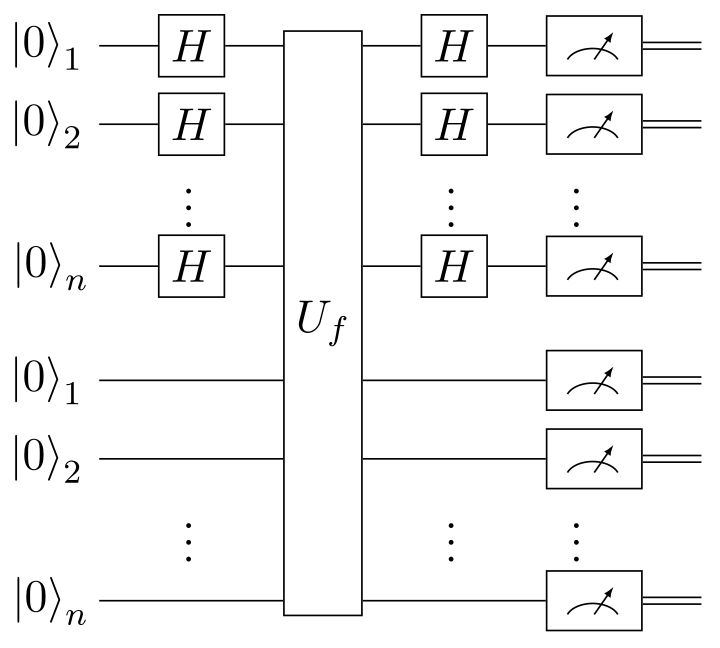Categories: Algorithms, Quantum information.

# Simon’s algorithm

Simon’s algorithm was the first proof that quantum computers are able to solve some problems exponentially faster than classical computers. In the same spirit as the Deutsch-Jozsa algorithm and the Bernstein-Vazirani algorithm, the problem it solves, known as Simon’s problem, is of no practical use, but nevertheless Simon’s algorithm is an important landmark.

Simon’s problem is this: we are given a “black box” function $$f(x)$$ that takes an $$n$$-bit input $$x$$ and returns an $$n$$-bit output. We are promised that there exists an $$s$$ such that for all $$x_1$$ and $$x_2$$:

\begin{aligned} f(x_1) = f(x_2) \quad \Leftrightarrow \quad x_2 = s \oplus x_1 \end{aligned}

In other words, regardless of what $$f(x)$$ does behind the scenes, its output is the same for inputs $$x_1$$ and $$x_2$$ if and only if $$x_2 = s \oplus x_1$$, or, equivalently, $$x_1 = s \oplus x_2$$.

The goal is to find the $$n$$-bit number $$s$$, using as few calls to $$f$$ as possible. There are two cases: if $$s = 0$$, then $$f$$ is one-to-one, since $$x_2 = 0 \oplus x_1 = x_1$$. Otherwise, if $$s \neq 0$$, then $$f$$ is two-to-one by definition: for every $$x_1$$ there exists exactly one $$x_2$$ such that $$x_2 = s \oplus x_1$$.

A classical computer solves this by randomly guessing inputs, until it finds two that give the same output, and then $$s = x_1 \oplus x_2$$. For $$n$$-bit numbers, this takes $$\mathcal{O}(\sqrt{2^n})$$ guesses (the square root is due to the birthday paradox).

A quantum computer needs to query $$f$$ only $$\mathcal{O}(n)$$ times, although the exact number varies due to the algorithm’s probabilistic nature. It uses the following circuit:The XOR oracle $$U_f$$ implements $$f$$, and has the following action for $$n$$-bit $$a$$ and $$b$$:

\begin{aligned} \ket{a} \ket{b} \quad \to \boxed{U_f} \to \quad \ket{a} \ket{b \oplus f(a)} \end{aligned}

Starting from the state $$\ket{0}^{\otimes 2 n}$$, we apply the Hadamard gate $$H$$ to each of the first $$n$$ qubits:

\begin{aligned} \ket{0}^{\otimes n} \ket{0}^{\otimes n} \quad \to \boxed{H^{\otimes n}} \to \quad \ket{+}^{\otimes n} \ket{0}^{\otimes n} = \frac{1}{\sqrt{2^n}} \sum_{x = 0}^{2^n - 1} \ket{x} \ket{0}^{\otimes n} \end{aligned}

Where $$\ket{x}$$ is shorthand for $$\ket{x}_1 \cdots \ket{x}_n$$. In other words, we now have an equal superposition of all possible inputs $$x$$, with a constant $$\ket{0}^{\otimes n}$$ beside it. We give this to the oracle $$U_f$$:

\begin{aligned} \frac{1}{\sqrt{2^n}} \sum_{x = 0}^{2^n - 1} \ket{x} \ket{0}^{\otimes n} \quad \to \boxed{U_f} \to \quad \frac{1}{\sqrt{2^n}} \sum_{x = 0}^{2^n - 1} \ket{x} \ket{f(x)} \end{aligned}

Then we apply $$H^{\otimes n}$$ to the first $$n$$ qubits again, which, thanks to the definition of the Hadamard transform, yields the following, where $$x \cdot y$$ is the bitwise dot product:

\begin{aligned} \frac{1}{\sqrt{2^n}} \sum_{x = 0}^{2^n - 1} \ket{x} \ket{f(x)} \quad \to \boxed{H^{\otimes n}} \to \quad &\frac{1}{2^n} \sum_{x = 0}^{2^n - 1} \bigg( \sum_{y = 0}^{2^n - 1} (-1)^{x \cdot y} \ket{y} \bigg) \ket{f(x)} % \\ % = &\sum_{y = 0}^{2^n - 1} \ket{y} \bigg( \frac{1}{2^n} \sum_{x = 0}^{2^n - 1} (-1)^{x \cdot y} \ket{f(x)} \bigg) \end{aligned}

Next, we measure all qubits. The order in which we do this does not matter, but, for clarity, let us measure the last $$n$$ qubits first, yielding $$\ket{f(x_1)}$$ for some $$x_1$$. Doing this leaves the $$2n$$ qubits in the following state, where $$f(x_1) = f(x_2)$$ and $$x_2 = s \oplus x_1$$:

\begin{alignedat}{2} &\mathrm{if} \: s = 0: \qquad &&\frac{1}{\sqrt{2^{n}}} \sum_{y = 0}^{2^n - 1} (-1)^{x_1 \cdot y} \ket{y} \ket{f(x_1)} \\ &\mathrm{if} \: s \neq 0: \qquad &&\frac{1}{\sqrt{2^{n+1}}} \sum_{y = 0}^{2^n - 1} \Big( (-1)^{x_1 \cdot y} + (-1)^{x_2 \cdot y} \Big) \ket{y} \ket{f(x_1)} \end{alignedat}

If $$s = 0$$, we get an equiprobable superposition of all $$y$$. So, when we measure the first $$n$$ qubits, the result is a uniformly random number, regardless of the phase $$(-1)^{x_1 \cdot y}$$.

If $$s \neq 0$$, the situation is more interesting, because we can only measure $$y$$-values where:

\begin{aligned} (-1)^{x_1 \cdot y} + (-1)^{x_2 \cdot y} \neq 0 \end{aligned}

Since $$x_2 = s \oplus x_1$$ by definition, we can rewrite this as follows:

\begin{aligned} (-1)^{x_1 \cdot y} + (-1)^{x_1 \cdot y \oplus s \cdot y} = (-1)^{x_1 \cdot y} + (-1)^{x_1 \cdot y} (-1)^{s \cdot y} \neq 0 \end{aligned}

Clearly, the expression can only be nonzero if $$s \cdot y$$ is even. In other words, when we measure the first $$n$$ qubits, we get a random $$y$$-value, for which $$s \cdot y$$ is guaranteed to be even.

In both cases $$s = 0$$ and $$s \neq 0$$, we measure a $$y$$-value that satisfies the equation:

\begin{aligned} s \cdot y = 0 \:\:(\bmod 2) \end{aligned}

This tells us something about $$s$$, albeit not much. But if we run Simon’s algorithm $$N$$ times, we get various $$y$$-values $$y_1, ..., y_N$$, from which we can build a system of linear equations:

\begin{aligned} s \cdot y_1 &= 0 \:\:(\bmod 2) \\ s \cdot y_2 &= 0 \:\:(\bmod 2) \\ &\:\:\vdots \\ s \cdot y_N &= 0 \:\:(\bmod 2) \end{aligned}

This can be solved efficiently by a classical computer. In the best-case scenario, all those $$y$$-values would be linearly independent (when regarded as vectors of bits), in which case only $$N = n - 1$$ equations would be necessary. Simon’s algorithm is therefore $$\mathcal{O}(n)$$.

It may feel like “cheating” to use a classical computer at the end. Remember that the point of this algorithm is to limit the number of oracle queries, which we did successfully. Querying an oracle might be a very expensive operation, so that is a big improvement! That said, Simon’s algorithm currently has no known practical uses.

1. J.S. Neergaard-Nielsen, Quantum information: lectures notes, 2021, unpublished.
2. S. Aaronson, Introduction to quantum information science: lecture notes, 2018, unpublished.